# Introduction

The focus of these notes is on inspecting and cleaning data before feeding it to models. The importance of this task is captured by the dictum, “Garbage in, garbage out”.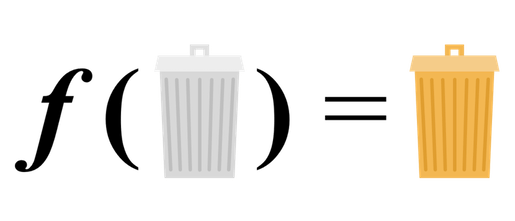All models have assumptions and limitations. “Garbage in” refers to inputting data that violates critical model assumptions, and “Garbage out” refers to the inaccurate results produced by feeding garbage to your models.

As data scientists, a HUGE part of our job is making sure we aren’t putting garbage into models. In fact, for many of is, this is the most time consuming part of our job!

The process of preparing data for modeling is so important that it deserves to be formalized, which brings us to a core concept of the course: The Inspect-Transform-Inspect workflow (ITI).

### Motivating example

Intuitively, linear regression draws a line through the “middle” of the data. Mathematically, the “middle” is usually defined as the line that minimizes the Sum of the Squared Errors (SSE), or the least squares line: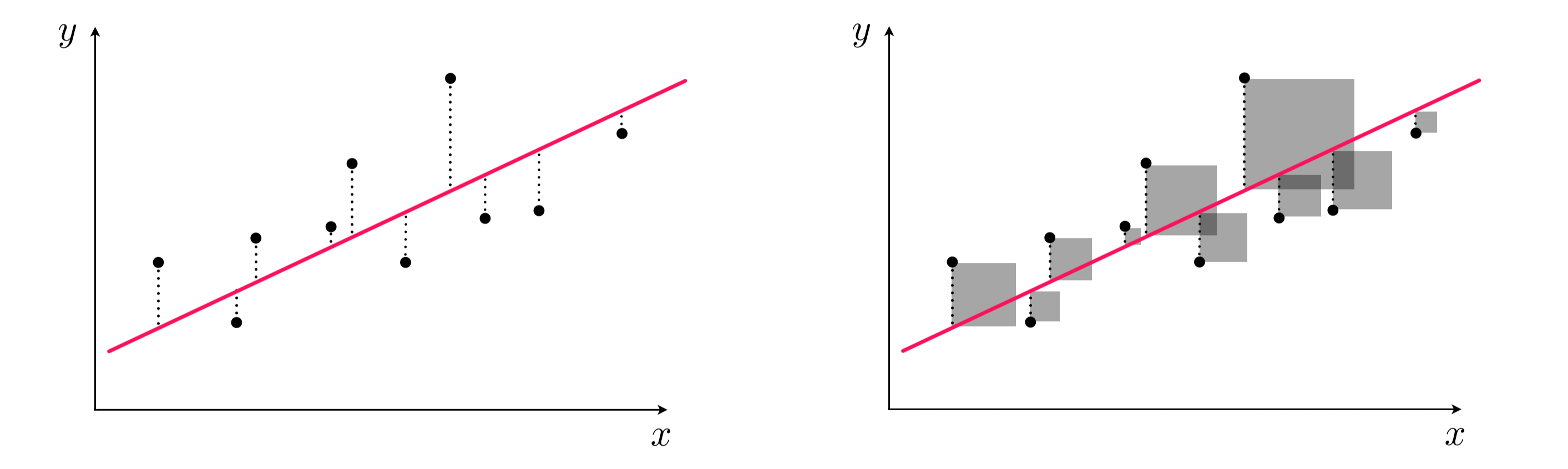When the errors are scattered evenly around the line (i.e., normally distributed errors), the least squares line produces the most accurate possible predictions. However, when the errors are NOT normally distributed, the least squares line can be misleading. Below, I simulate some data with outliers and skew to demonstrate two common ways in which non-normal errors can confuse linear regression:

``````library('tidyverse')
library('gridExtra')

set.seed(23)
n <- 100

# Simulate normally distributed x and y
normal_x <- rnorm(n)
y <- normal_x + rnorm(n, sd=0.25)

# Outlier version of x
outlier_x <- normal_x
outlier_x[which(outlier_x==min(outlier_x))] <- outlier_x[which(outlier_x==min(outlier_x))] + 10

# Skewed version of x
skewed_x <- exp(normal_x)

# Combine variables into dataframe
df <- data.frame(y, normal_x, outlier_x, skewed_x)

# Plot
p1 <- df %>% ggplot(aes(normal_x, y)) + ylim(-3, 3) +
geom_point() + geom_smooth(method='lm') + ggtitle('Good')
p2 <- df %>% ggplot(aes(outlier_x, y)) + ylim(-3, 3) +
geom_point() + geom_smooth(method='lm') + ggtitle('Bad - outlier')
p3 <- df %>% ggplot(aes(skewed_x, y)) + ylim(-3, 3) +
geom_point() + geom_smooth(method='lm') + ggtitle('Bad - nonlinearity (skew)')

# Plot linear regressions
grid.arrange(p1, p2, p3, nrow=1)``````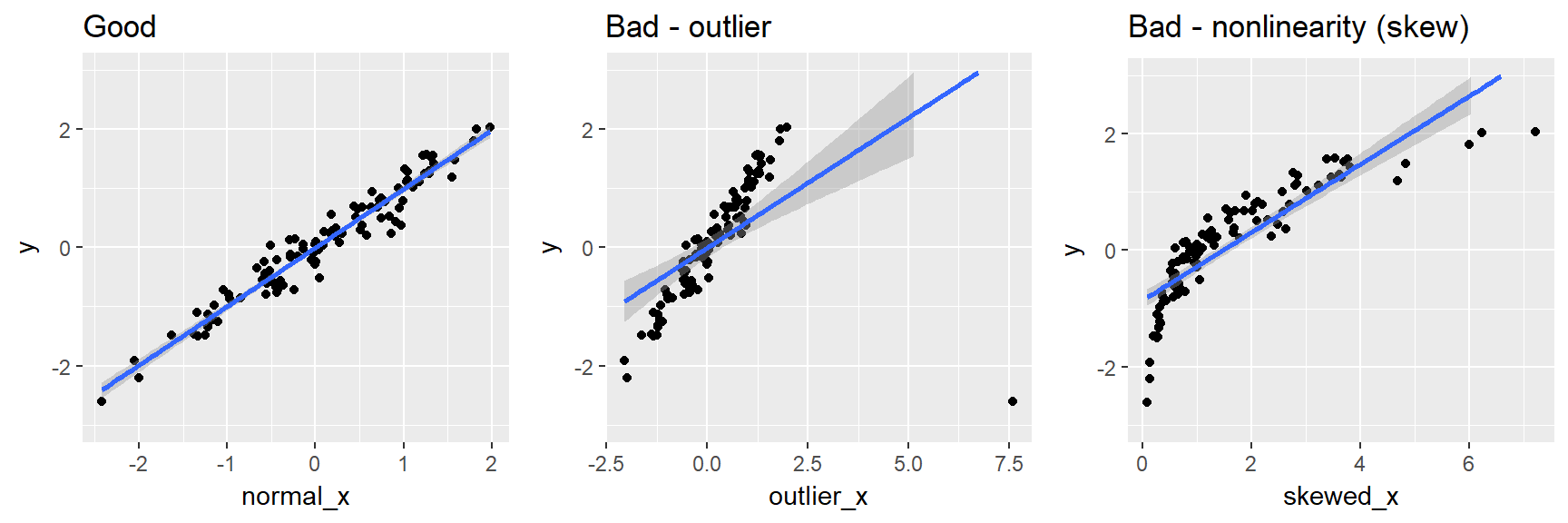The first plot above shows a ‘healthy’ linear regression of `y ~ x`, where both `y` and `x` are normally distributed. The middle plot shows what happens to the line if we insert a single severe outlier into `x`, and the plot on the far right shows what happens if we force `x` to be skewed by exponentiating it.

Notice how in both the middle and right plots, the line does not go through the ‘middle’ of the data and the points are not scattered evenly around the line. If we were to use these models to make predictions, they would be extremely biased and inaccurate!

THIS is why we must inspect and transform our data before feeding it into our models. In this case, since we have continuous features, we can inspect them individually with histograms:

``````# Plot histograms of the different versions of x
p1 <- df %>% ggplot(aes(x=normal_x)) + geom_histogram(bins=9)
p2 <- df %>% ggplot(aes(x=outlier_x)) + geom_histogram(bins=9)
p3 <- df %>% ggplot(aes(x=skewed_x)) + geom_histogram(bins=9)
grid.arrange(p1, p2, p3, nrow=1, top="Original predictor variables")``````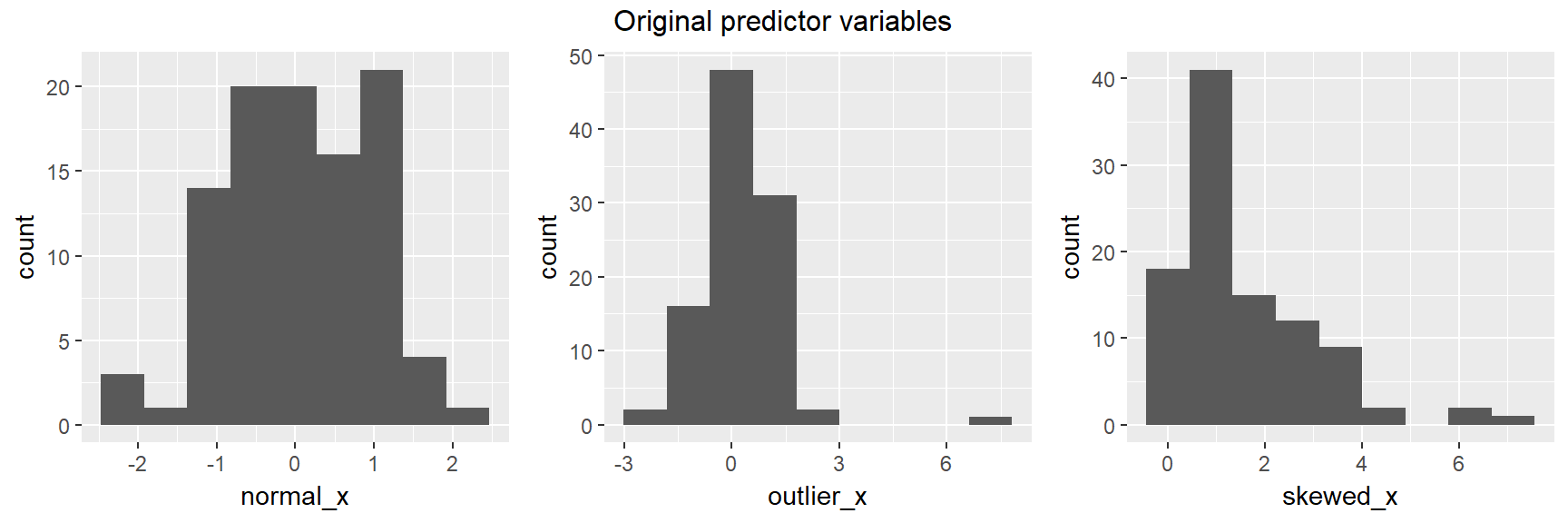Now, we can fix the problems with some standard variable transformations and plot the histograms again to show that the variables are ‘fixed’ (don’t worry, we will discuss these transformations shortly):

``````# Drop outliers
df <- df %>% filter(abs(outlier_x) < 5)

# Log tansform the skewed variable
df\$skewed_x <- log(df\$skewed_x)

# Plot histograms of the transformed versions of x
p1 <- df %>% ggplot(aes(x=normal_x)) + geom_histogram(bins=9)
p2 <- df %>% ggplot(aes(x=outlier_x)) + geom_histogram(bins=9)
p3 <- df %>% ggplot(aes(x=skewed_x)) + geom_histogram(bins=9)
grid.arrange(p1, p2, p3, nrow=1, top="Transformed predictor variables")``````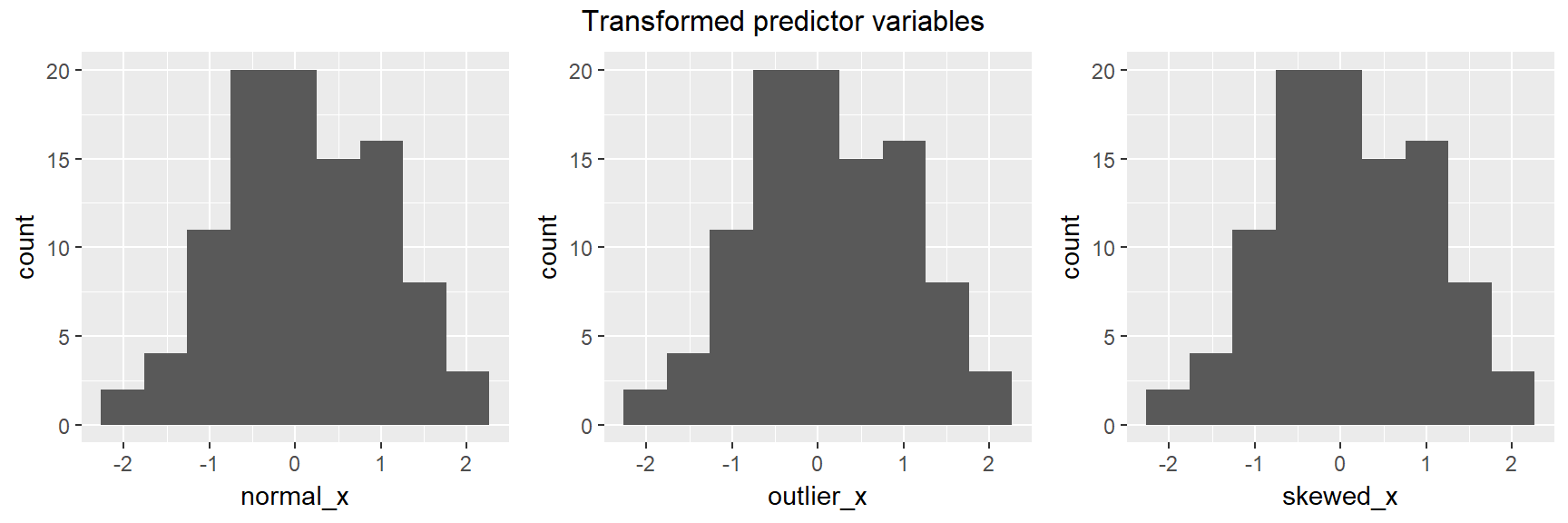They all look relatively normal now. Now we can refit the linear regressions on the transformed data and observe that all 3 appear to be healthy linear regressions:

``````# Plot
p1 <- df %>% ggplot(aes(normal_x, y)) +
geom_point() + geom_smooth(method='lm') + ggtitle('Good')
p2 <- df %>% ggplot(aes(outlier_x, y)) +
geom_point() + geom_smooth(method='lm') + ggtitle('Good')
p3 <- df %>% ggplot(aes(skewed_x, y)) +
geom_point() + geom_smooth(method='lm') + ggtitle('Good')

# Plot linear regressions
grid.arrange(p1, p2, p3, nrow=1)``````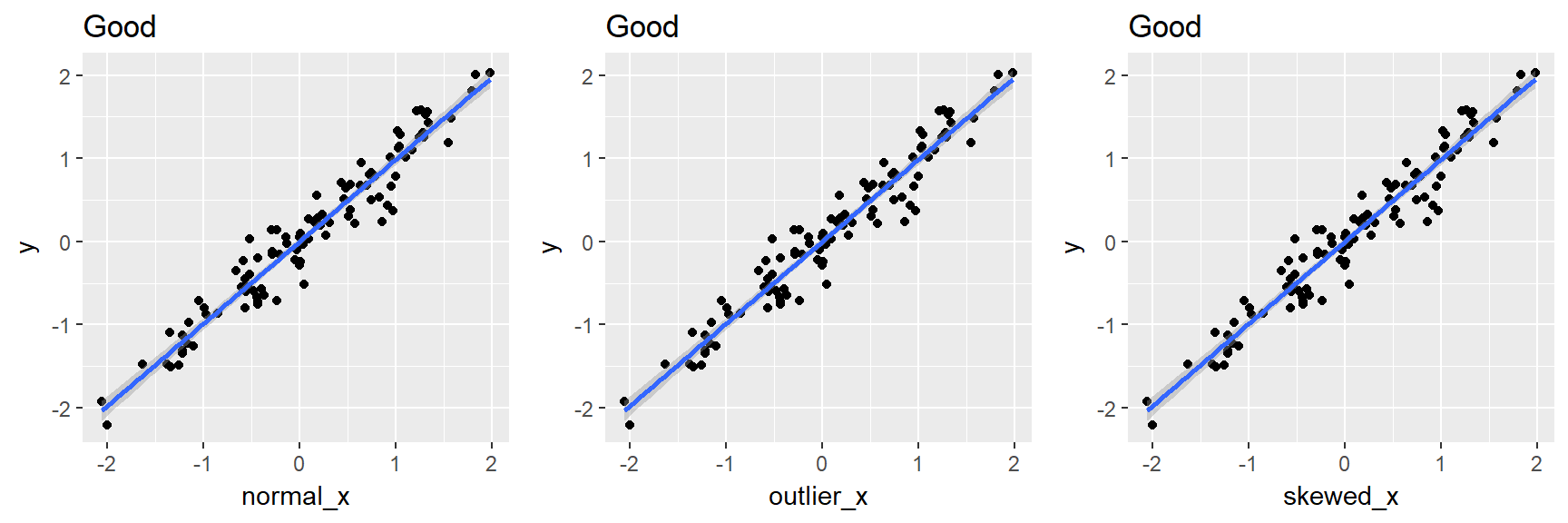Much better! In the next section, we will generalize the process that unfolded above to other types of variables.

### Inspect-Transform-Inspect workflow (ITI)

The ITI workflow is an iterative process in which you inspect and transform your variables until they are ‘clean’. This messy process is almost always hidden from view in the final version of a data science project, but in reality, it occupies much of your time as an analyst and has a huge impact on the quality of your results. This is the unglamorous part of scientific research in which you painstakingly set up your experiment and obsess over details that could mislead your results: